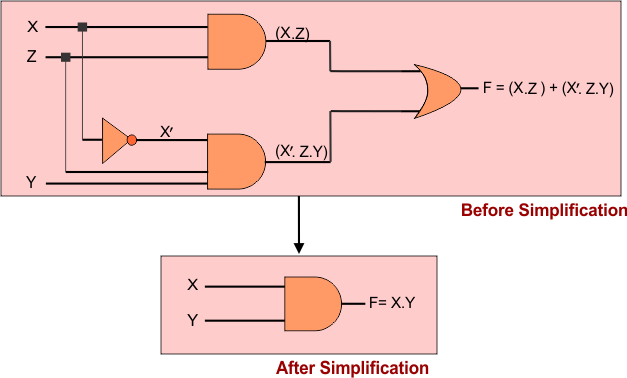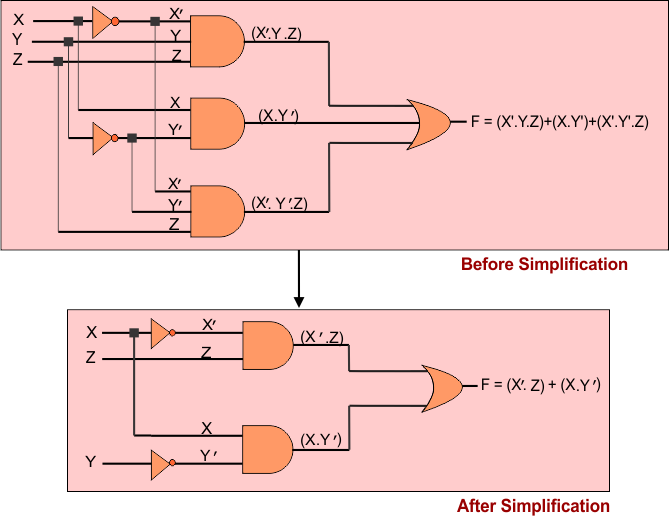Select Page

Computer Organization

Memory Organization
Interrupt
DMA Controller

# Simplify Boolean Expressions Using Laws

Boolean Expressions can easily Simplify by Using Laws of Boolean Algebra. Let explain some examples to Simplify Boolean Expressions.

## Example 01

F(X, Y, Z) = X.Z+X’.Z.Y

F(X, Y, Z) = X.Z+X’.Y.Z     {By Commutative and commutative law}

F(X, Y) = (X+X’.Y).Z         {By Distributive law}

F(X, Y) = (1.Y).Z              {by complement law}

F(X, Y) = (X.Y)                 {by definition of identity element}

Hence, the simplified Boolean function will be X.Y

### Symbolically Simplification

Logic diagram, before and simplification for the Boolean function F(X, Y, Z) = X.Z+X’.Z.Y is given below## Example 02

F(X, Y, Z) = X’.Y.Z+X.Y’+X’.Y’.Z

F(X, Y, Z) = X’.Y.Z + X’.Y’.Z + X.Y’ {By Commutative law}

F(X, Y, Z) = X’.Z(Y+Y’) + X.Y’  {By Distributive law}

F(X, Y, Z) = X’.Z (1) + X.Y’ {by complement law}

F(X, Y, Z) = X’.Z + X.Y’ {by definition of identity element}

Hence, the simplified Boolean function will be X’.Z + X.Y’

### Symbolically Simplification

Logic diagram, before and simplification for the Boolean function F(X, Y, Z) = X’.Y.Z+X.Y’+X’.Y’.Z is given belowHelp Other’s By Sharing…